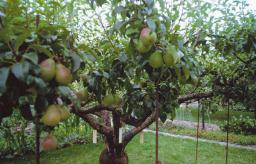# Quarter 3106

In the orchard, there were 46 more apple trees than pears. The storm upset a quarter of apple trees and seven pears; however, there were still 80 trees left. How many were apple trees and how many pears in the garden?

a =  76
b =  30

### Step-by-step explanation:

a=46+b
a/4+7=a+b-80

a-b = 46
3a+4b = 348

Pivot: Row 1 ↔ Row 2
3a+4b = 348
a-b = 46

Row 2 - 1/3 · Row 1 → Row 2
3a+4b = 348
-2.33b = -70

b = -70/-2.33333333 = 30
a = 348-4b/3 = 348-4 · 30/3 = 76

a = 76
b = 30

Our linear equations calculator calculates it.Did you find an error or inaccuracy? Feel free to write us. Thank you!

Tips for related online calculators
Do you have a system of equations and looking for calculator system of linear equations?